Mathematical Modelling and Simulation of Fouling of Nigerian Crude Oil Equipment Installations

Abdulsalami S. KOVO

Department of Chemical Engineering, Federal University of Technology, Minna, pmb 65, Nigeria,

Abstract

The aim of this research work was to develop Mathematical Model to Predict the Fouling of Nigeria Oil Equipment Installation. The term fouling can defined as the deposition of unwanted material on heat transfer equipment, which results in an increase in thermal resistance to heat transfer and subsequent loss of equipment thermal efficiency. The modelling equation formulated was based on the principle of fouling process being in unsteady state condition. That is, the rate of deposition is not equal to the rate of removal. In other words, there is an accumulation. The final mathematical model developed representing the fouling of Nigeria Oil equipment was obtained as: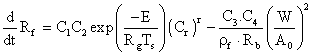. The correlation between the experimental and simulated result obtained using Average Absolute Deviation (A.A.D) was between 0.0131 - 0.0201. The fluid temperature of 43°C and 63°C and wire temperature 200°C, 250°C and 300°C were the operating conditions for the investigation and prediction. The model was simulated using VISUAL BASIC 6.0 to predict the fouling behaviour. The results obtained showed that refinery Naptha fouls very heavily and this fouling decreases with increasing fluid and wire temperature. Fouling was very severe for wire temperature 200°C and fluid temperature 46°C. The main mechanism of fouling for refinery Naptha was chemical polymerization.

Keywords

Fouling rate, Mathematical model, Visual Basic Programme, Crude oil, Average Absolute Deviation, Fluid Temperature, Wire Temperature

Introduction

The term fouling is defined as the deposition of unwanted material on heat transfer equipment, which results in an increase in thermal resistance to heat transfer and subsequent loss of equipment thermal efficiency. [Bott, 1995]. This deposition includes organic reaction products, high molecular weight process stream components, coke and inorganic salts, involving the transfer of heat either from a fluid to the solid of vice versa.

Crude oil fouling is generally behaved to be caused by impurities present in the crude oil such as corrosion product (iron sulphide and rust), water and salt sodium chloride calcium chloride and magnesium chloride] by asphateries exceeding their solubility limits, or by thermal decomposition or autoxidation of reactive constituents in the oil. Aspaltenes precipitation is considered to be a major cause of crude unit fouling [Murphy and Campbell, 1992].

At the same time, reactive constituents or oil may under thermal decomposition, polymerization, or autoxidation reaction to produce fouling precursors of foulant.

Dickakian (1997) has shown the role of asphaltenes and polar molecules in fouling at elevated temperature where coke is produced following phase separation. At lower temperatures, asphaltenes precipitation occurs due to changes in solvent nature via blending or pressure change.

Fouling leads to reduce heat transfer, increase pressure drop, blocked process pipes, and these effects are costly. The cost factors include higher heat exchanger requirements, increase fuel consumption, reduce throughput and increase maintenance. In addition to the operation losses, fouling force significant capital expenditure, the fouling factors are taken into consideration.

Fouling phenomenon can also be experienced in gas-solid processes, such as deposition of carbonacuoles particles, oil and in combustible on the exhaust pipes of automobiles, fuel- powered engines and chimneys. Liquid-solid fouling also is found in obnoxious particle deposition in process flow lines, public water supply pipes, and the fouling of domestic cooking. Fouling  on utensils e.g kettles, make fouling  a menace not only to the petroleum industry but also at home pots and pans had been a domestic nuisance which at times need such drastic measure like scrapping off the deposit with knife edges.

Mathematical modelling is a comprehensive method of representing a physical phenomenon, in terms of mathematical equation while computer simulation is the use of computer to generate solution to a problem or the behaviour of a system. [Meyer, 1992]. The utilization of basic laws of physics and chemistry are used to describe mathematically the operations of a reactor. The mathematical model developed, generally consist of a set of differential equations that are frequent too complex, that they become impossible to solve by analytical methods. However, the solution of the mathematical model derived, can often be accomplished by simulation of the model with an analogue or a digital computer. The success of the approach is governed not only by the completeness and reliability of the mathematical model but also by the amount and accuracy of the basic experimental data [Perry and green 1997]. The objective of this paper is to develop a model equation to predict fouling rate of Nigerian crude oil equipment installations. The fouling characteristics of oil equipment installation are influenced by such fouling factor as rate of fouling, effect of certain process variable such as flow rate, temperature of bulk and surface, oxygen concentrations on the rate or degree of fouling. This process variable will be incorporated in the development of the final model.

Experimental Procedure

All the bolts, elbows and seals were checked for any leaking points. The 2.5 gallon tank was filled with Naphtha fluid heated up by an immersion heater. The fluid temperature in the tank was measured by mercury in glass thermometer. The fluid was pumped via a back-mix with value V2 and V4 closed while V1 and V3 were opened as obvious from figure 1. This was allowed to operate for 20 minutes while at the same time adjusting the cooling water valve to ensure that a constant temperature desired was achieved in the tank. When the temperature is fairly constant indicated by the thermometer reading valve V3 was shut while valve V2 and V4 were opened. The variance switch was put on and adjusted to the current that gives the desired wire temperature.

The fluctuation in the wire resistance was basically due to move touching the pipe. This was being read corresponded to that which gave the same power dissipated. This was achieved by measuring the wire resistance from (V/I) and evaluating the power (I2R) to see whether it is within the operating power range, the system was allowed to equilibrate to steady state by allowing 15 minutes to elapse prior commencement of measurement. After 15 minutes, the inlet and outlet potentiometer reading was taken.

The manometer and gauge reading were noted. The ambient temperature was also taken. Subsequent readings were taken after one hour voltage reading was checked at interval for any fluctuations. Stirring was done manually and the fluid temperature was basically controlled by the cooling water into the heat exchanger and intermittently switching on and off of the immersion heater.

Conceptualization of Modelling Technique -DIAGRAM

Taking for instance that there is a channel (pipe) through which oil is flowing, assuming that the inner part of the pipe is open as shown in figure 1 below, the fouling process that takes in the pipe can be depicted as given below.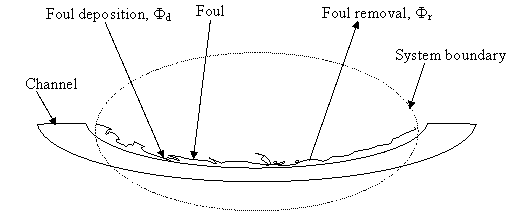Figure 1. Schematic representation of fouling process

Assumptions

In the course of modelling of the fouling characteristics of Nigerian crude oils, the assumptions made include:

(i)            The fouling process is an unsteady one. That is, the rate of deposition is not equal to the rate of removal.  In other words, there is accumulation.

(ii)          The deposition is reaction rate controlled.

(iii)         The flow rate of the liquid (crude oil) is constant.

Model formulation

Starting from the basic principle of mass balances which states that

Input = Output + Accumulation                                                                         (1)

That is, Rate of deposition = rate of removal + foul accumulation

Mathematically:

Rate of deposition = d

Rate of removal = r

Foul accumulation is given as the rate of change of foul and is denoted, mathematically, as

Considering equation 1 (Rate of deposition = Rate of removal + Foul accumulation rate), substituting the various terms yields: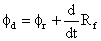(2)

Making the accumulation term the subject of the formula gives: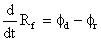(3)

Taborek et al (1972), from their numerous observations, found out that some typical behaviour patterns can be rationalized. In principle, the net fouling rate of crude oil can be expressed as the difference between a deposition and a removal rate equation described by the mathematical relation given below as:(4)

where Rf is the net fouling rate, Fd is the rate of deposition, and Fr is the rate of removal.

Using the reaction controlled deposition, according to assumption No. 2, Taborek et al (1972) has proposed that: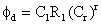(5)

where Cr is the concentration of the material undergoing the reaction, r is the reaction order

Rr is the reaction rate constant defined as (Rectfer, 1964):

Rr = C2·e[(-E)/(RgTg)]                                                                                            (6)

where C2 is the proportionality constant, E is the activation energy, and Rg is the universal gas constant.

It should be noted that the exponential temperature relationship of this type of deposition mechanism requires careful attention to the effect of design wall temperatures on deposition rates and eventually maintenance costs.

Substituting the relationship of the Rr into the equation of Fd gives:(7)

Further, the rate of removal is given as: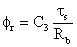(8)

where ts and Rb are the fluid shear and deposition bond resistance respectively, and C3 is a constant.

In addition, ts is given as (Brawn & Hansler, 1976):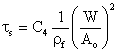(9)

It should be noted that C4 is also a constant. Now, substituting equation (9) into (8)(10)

which is the same as:(11)

where W is the flow rate of liquid which is constant (according to assumption No. 3), Ao is the cross sectional area of the flow of the crude, and rf is the density of the crude oil.

Now, the net fouling rate which was originally given as:(12)

can now be written as, after substituting the relationships of Fd and Fr:(13)

In other words,(14)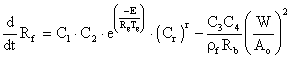(15)

The equation above is the model for the fouling rate of Nigerian crude oils.

Results and Discussion

Experimental and simulated results obtained for the Run 1-5 modelling express as fouling rates for, errors and standard deviations according with the time are in table 1-5, and graphical represented in figures 2-5.

Table 1. Fouling Rates (cal/hr cm2 °C) for Run 1

 Time (hr) Experimental Simulated Error Std. Dev. 0 0.00 0.00 0.00 0.0000 1 33.27 33.26 0.01 0.0071 2 223.84 223.81 0.03 0.0212 3 44.38 44.39 -0.01 0.0071 4 95.55 95.55 0.00 0.0000 5 172.68 172.84 -0.16 0.1131 6 286.07 286.10 -0.03 0.0212 7 290.15 290.10 0.05 0.0354 8 442.77 442.79 -0.02 0.0141 9 712.95 712.96 -0.01 0.0071 10 1285.78 1285.76 0.02 0.0141 11 1226.41 1226.42 -0.01 0.0071 12 2774.12 2774.10 0.02 0.0141 Average Absolute Deviation = 0.0201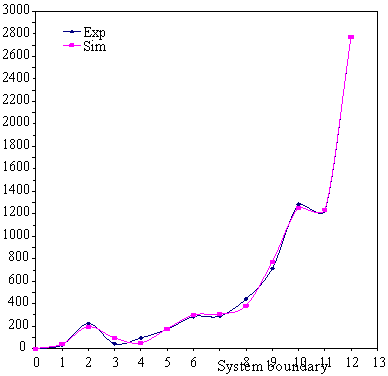Time (hr)

Figure 2. Graphs of experimental and simulated fouling rates for Run 1 using statistical method (TFL = 46°C, Tw = 200°C)

Table 2. Fouling Rates (cal/hr cm2 °C) for Run 2

 Time (hr) Experimental Simulated Error Std. Dev. 0 0.00 0.00 0.00 0.0000 1 0.00 0.00 0.00 0.0000 2 0.00 0.01 -0.01 0.0071 3 2.59 2.57 0.02 0.0141 4 32.40 32.41 -0.01 0.0071 5 42.21 42.23 -0.02 0.0141 6 89.44 89.42 0.02 0.0141 7 151.81 151.84 -0.03 0.0212 8 241.40 241.38 0.02 0.0141 9 358.41 358.31 0.10 0.0707 10 89.44 89.46 -0.02 0.0141 11 531.85 531.89 -0.04 0.0283 12 1131.53 1131.55 -0.02 0.0141 Average Absolute Deviation = 0.0169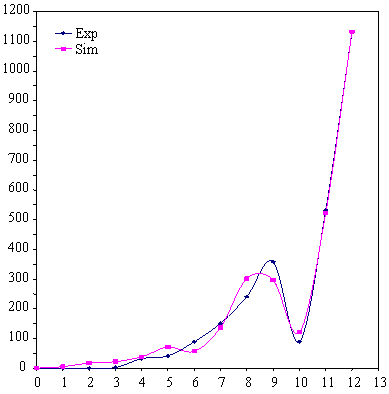Time (hr)

Figure 3. Graphs of experimental and simulated fouling rates for Run 2 using statistical method (TFL = 46°C, Tw = 250°C)

Table 3. Fouling Rates (cal/hr cm2 °C) for Run 3

 Time (hr) Experimental Simulated Error Std. Dev. 0 0.00 0.00 0.00 0.0000 1 2.55 2.54 0.01 0.0071 2 0.00 0.02 -0.02 0.0141 3 34.75 34.79 -0.04 0.0283 4 34.75 34.74 0.01 0.0071 5 53.96 53.94 0.02 0.0141 6 73.31 73.29 0.02 0.0141 7 77.03 77.05 -0.02 0.0141 8 71.48 71.47 0.01 0.0071 9 120.32 120.34 -0.02 0.0141 10 178.89 178.91 -0.02 0.0141 11 353.35 353.32 0.03 0.0212 12 506.20 506.18 0.02 0.0141 Average Absolute Deviation = 0.0131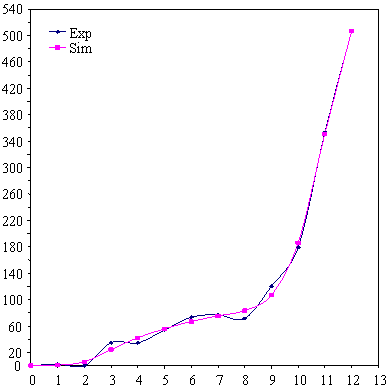Time (hr)

Figure 4. Graphs of experimental and simulated fouling rates for Run 3 using statistical method (TFL = 46°C, Tw = 300°C)

Table 4. Fouling Rates (cal/hr cm2 °C) for Run 4

 Time (hr) Experimental Simulated Error Std. Dev. 0 0.00 0.00 0.00 0.0000 1 3.87 3.86 0.01 0.0071 2 9.16 9.16 0.00 0.0000 3 57.80 57.78 0.02 0.0141 4 27.47 27.46 0.01 0.0071 5 14.60 14.60 0.00 0.0000 6 47.72 47.77 -0.05 0.0354 7 70.21 70.17 0.04 0.0283 8 142.86 142.83 0.03 0.0212 9 145.37 145.38 -0.01 0.0071 10 271.79 271.80 -0.01 0.0071 11 275.77 275.74 0.03 0.0212 12 490.31 490.36 -0.05 0.0354 Average Absolute Deviation = 0.0141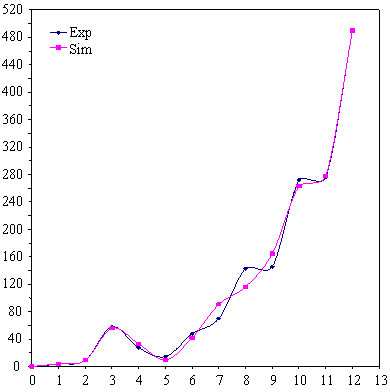Time (hr)

Figure 5. Graphs of experimental and simulated fouling rates for Run 4 using statistical method (TFL = 63°C Tw = 200°C)

Table 5. Fouling Rates (cal/hr cm2 °C) for Run 5

 Time (hr) Experimental Simulated Error Std. Dev. 0 0.00 0.00 0.00 0.0000 1 0.00 0.00 0.00 0.0000 2 39.94 39.91 0.03 0.0212 3 1.44 1.45 -0.01 0.0071 4 2.91 2.93 -0.02 0.0141 5 0.00 0.01 -0.01 0.0071 6 45.30 45.26 0.04 0.0283 7 41.71 41.72 -0.01 0.0071 8 107.58 107.56 0.02 0.0141 9 194.49 194.44 0.05 0.0354 10 194.49 194.51 -0.02 0.0141 11 309.43 309.45 -0.02 0.0141 12 309.43 309.44 -0.01 0.0071 Average Absolute Deviation = 0.0131

In the experimentations and simulation result, the Progressive decrease of heat transfer coefficient obviously, shows that fouling had taken place. This trend was not however, uniform, which is expected for chemical-polymerization of fouling of hydrocarbon streams. This reveals that other mechanisms of fouling superimposed on chemical polymerization fouling. Nevertheless chemical polymerization fouling was observed to take place by the gummy-brown colouration of Nichrome wire. A cross sectional looks in the tank and fluid stream shows chemical reaction taking place. This is obvious since the golden-oil colour of Naptha becomes milky at first which then turns rapidly brown. The lower heat transfer coefficient at the operating run of Tw = 200°C and TFL = 46°C compared to subsequent runs of the same fluid but different wall or wire temperature after the 12th hours of run shows severe fouling at this condition. As the wire temperature increases for the same fluid temperature the heat transfer coefficient moves up.

In Figure 3 for wall temperature Tw = 250°C and TFL = 46°C after 5 runs shows  uniformly decreasing heat transfer coefficient with time, which indicate chemical polymerization fouling for this period. To achieve the same fouling as to when wire temperature was Tw = 200°C and TFL = 46°C for higher wire temperature, more time must be allowed. Hence, the amount of fouling that can occur is only in a matter of time, irrespective of the operating condition to give the same problem.

The undulating and oscillatory trend of fouling rate with time for fluid temperature of 63°C obviously shows another fouling behaviour at this temperature which was shown in figure 4 and 5.

The fouling resistance Rf with time is highest for a wire temperature of 200°C and least for wire temperature 300°C after 12 hours. The fouling resistance for the 4hours period of run reveal a situation where the wire temperature is activated for fouling shown in Table 5. The wire temperature of 300°C plot of RF with time shows creeping trend. Nonetheless the fouling resistance with time after the 4th hour shows a non-uniformly upward increasing tend revealing the unpredictive nature of fouling behaviour.

The induction period is more pronounced in the plot of DRf/Dt with time. The plot from figure 4 shows that for wire temperature of 300°C, the rate of fouling gradually increase almost constantly for the 8 hours of run showing that the rate of fouling at this temperature has decreased.

However, the fouling rate behaviour at Tw =200°C shows an unusual trend. The fouling rate decrease non-uniformly with time until after 7 hours of run, after which the fouling resistance rate increases uniformly with time and decreases again (figure 2). The overlapping nature of the curves of wire temperature at 200°C and 250°C (figure 2 and 3) reveal complex fouling behaviour at this fluid temperature of 63°C. The deposition of particles on the wire was obvious after the end of the experiment. It was observed that the Nichrome wire was covered with foul of brownish material.

Conclusions

The following conclusions can be made from the discussion and results:

1.      The main mechanism of fouling operative in the system is chemical reaction polymerization super imposed with other fouling mechanism.

2.      The fouling of refinery Naptha reveals a period of induction to activate the surface for chemical reaction.

3.      The fouling is most severe with fluid temperature of 46°C and wire temperature 200°C (figure 5).

4.      The fouling rate diminishes for wire temperature above 200°C.

5.      At a fluid temperature of 63°C refinery Naptha fouls less and a different fouling behaviour is observed (Figure 2 and 3).

6.      The overlapping nature of the curves for fluid temperature of 63°C and wire temperature of 200°C and 250°C for fouling rate with time shows a complex nature of fouling behaviour irrespective of which operating wire temperature (figure 2 and 3).

References

 Brawn R., Hanster R.H., Contribution to the Understanding of Fouling Phenomenon in Petroleum Industry, Paper Presented at the 16th National Heat Transfer Conference St Louis, Missouvi, 1976.

 Brawn R., The Nature of Petroleum Fouling - Results with Practical Instruments, Material Performance 1977, 16(11), p. 35.

 Hansler R. H., Fouling Studies on Hydrocarbon Streams in the Petroleum Industry, Paper Presented at the National Association of Corrosion Engineer J - 8 - 2, Committee on Refinery Corrosion, Chicago, 1973.

 Irwin A. Wielie, The Chemistry of Petroleum Fouling, Paper Presented at Spring AIChE Meeting on April, 2001.

 Kerm D. Q., Seaton R. E., A Theoretical Analysis of Thermal Surface Fouling, Paper Presented at the Symposium of Air Cooled Heat Exchangers, 51st Annual Meeting Cincinnat Dec., 1958.

 Luyben W. L., Process Modelling Simulation and Control for Chemical Engineers, 2nd Edition, McGraw Hill, Singapore, pp. 17 -124, 1995.

 Meyers R. A., Encyclopaedia of Physical Science and Technology, 2nd Edition, Volume 14, Academic Press Inc. London, pp. 348 - 369, 1992.

 Meyers R. A., Encyclopaedia of Physical Science and Technology, 2nd Edition, Volume 9; Academic Press Inc. London, pp. 519 - 528, 1992.

 Perry R. H., Green D., Perry’s Chemical Engineers Handbook, 6th Ed., McGraw Hill Publishing Company, Singapore, 1984.

 Rectfer B.J., Rate of Scale Formation in Tabular Heat Exchanger, 1 & EC Process Design and Development, 1964, 3(4), p. 345.

 Report of Work Group T - 8 - 29 (Refinery Industry Corrosion, Chicago Area), Issue by NACE Group Committee T - 8 on Refining Industry Corrosion Material Protection, 1970, 9, p. 29.

 Taborek J. et al., Predictive Methods of Fouling Behaviour, Chem Engr Program, 1972, 68(7), p. 69.

 Watkinson A. P., Epstein N., Chemical Engineering Program, Symposium Ser. 65, No. 92, 1969.